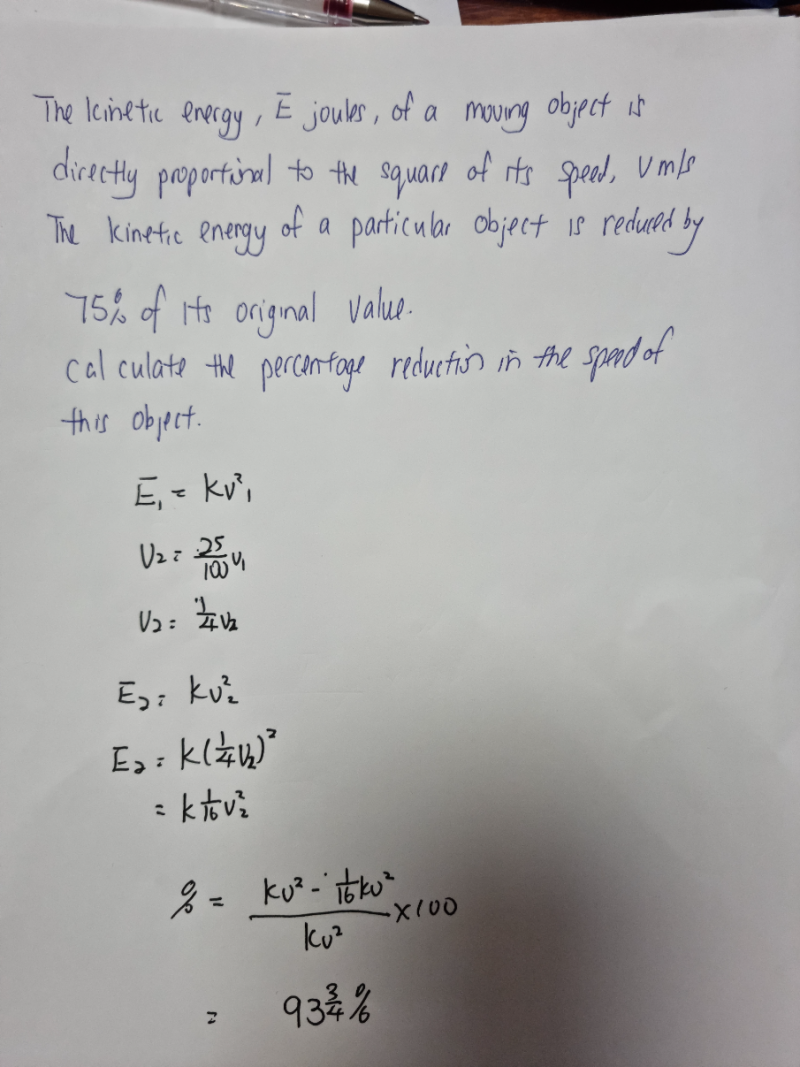# QuestionIs my steps correct.pls help .thank you.

It is incorrect because the question says the KE is reduced by 75% but your working shows that you have reduced  speed by 75% .   The reduction % in speed is what you need to find.  Instead your end result is finding the % decrease in the KE.

Information from first line gives you:

KE = (1/2) mv2     (formula for kinetic energy and it shows the direct proportion relationship, ie,

when speed v increases, v increases, KE increases, when v decreases, v2

decreases, KE decreases.)

So second line says KE decreases by 75% of its original value,

This means new KE = 0.25 (1/2) mv2.   ( (1/2)m is a constant, so you do not have to bother about it)

=> New KE = (0.5v)2 (1/2)m

=> New speed has decreased from v to 0.5v

So  %  of reduction in v is  [(v-0.5v) / v] x 100% = 50%

The first attempt in the earlier solution, I made a mistake of skipping a step…so I took the reduction in v2  instead of v = speed.

0 Replies 1 Like